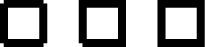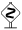0.5 KB
7.5 KB
0.6 KB
0.8 KB
0.6 KB```from pyx import *

unit.set(wscale=10)

c = canvas.canvas()

c.stroke(path.line(0, 0, 1, 0) +
path.line(1, 0, 1, 1) +
path.line(1, 1, 0, 1) +
path.line(0, 1, 0, 0))

c.stroke(path.line(2, 0, 3, 0) <<
path.line(3, 0, 3, 1) <<
path.line(3, 1, 2, 1) <<
path.line(2, 1, 2, 0))

p = path.line(4, 0, 5, 0) << path.line(5, 0, 5, 1) << path.line(5, 1, 4, 1)
p.append(path.closepath())
c.stroke(p)

```

### Description

The result of this example looks the same as the pathitem example from the Basic drawing section. However, we are using a different approach to construct the paths in this example: we add and join paths. When adding two paths, the resulting path just contains all the subpaths from the first and the second path as its subpaths. The join operation between two paths is similar but it combines the last subpath from the first path and continues it by the first subpath of the second path. Thus, join does not increase the number of subpaths like the add operation.A similar result in terms of the line thickness could be achieved by setting a different scale for the linewidth by

```unit.set(wscale=10)
```

immediately after the import statement. The unit system of PyX is aware of several length types (unscaleable true coordinates `t`, regular user coordinates `u`, visual coordinates `v` for arrows, symbols and other small visual components, line widths `w` and the text sizes `x`). The length types can be scaled independent of each other (except for true coordinates, which cannot be scaled at all).Note that joining two paths might insert a line connecting the first and the second path. For example, we could skip the second line when constructing `rect2` in our example above without any change in the result.